# Importance Simulation¶

Let us note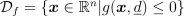. The goal is to estimate the following probability:This is a sampling-based method. The main idea of the Importance Sampling method is to replace the initial probability distribution of the input variables by a more “efficient” one. “Efficient” means that more events will be counted in the failure domain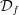and thus reduce the variance of the estimator of the probability of exceeding a threshold. Let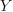be a random vector such that its probability density function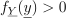almost everywhere in the domain,The estimator built by Importance Sampling method is: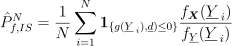where:

•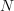is the total number of computations,

• the random vectors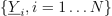are independent, identically distributed and following the probability density function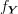Confidence Intervals

With the notations,The asymptotic confidence interval of order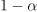associated to the estimator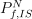is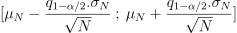where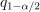is the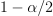quantile from the standard distribution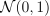.

This method could also be found under the name “Strategic Sampling”, “Weighted Sampling” or “Biased Sampling” (even if this estimator is not biased as it gives exactly the same result).